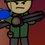# I don't understand just why

so apparently 0 divided by 0 is just undefined. I don't understand at all why, seeing that its pretty much just everything. My opinion is that 0 divided by 0 is actually any number, that there is no wrong answer, only correct ones. But I'm not a professional, so somebody help me with this.Note by Odin Wang
9 months ago

This discussion board is a place to discuss our Daily Challenges and the math and science related to those challenges. Explanations are more than just a solution — they should explain the steps and thinking strategies that you used to obtain the solution. Comments should further the discussion of math and science.

When posting on Brilliant:

• Use the emojis to react to an explanation, whether you're congratulating a job well done , or just really confused .
• Ask specific questions about the challenge or the steps in somebody's explanation. Well-posed questions can add a lot to the discussion, but posting "I don't understand!" doesn't help anyone.
• Try to contribute something new to the discussion, whether it is an extension, generalization or other idea related to the challenge.

MarkdownAppears as
*italics* or _italics_ italics
**bold** or __bold__ bold
- bulleted- list
• bulleted
• list
1. numbered2. list
1. numbered
2. list
Note: you must add a full line of space before and after lists for them to show up correctly
paragraph 1paragraph 2

paragraph 1

paragraph 2

[example link](https://brilliant.org)example link
> This is a quote
This is a quote
    # I indented these lines
# 4 spaces, and now they show
# up as a code block.

print "hello world"
# I indented these lines
# 4 spaces, and now they show
# up as a code block.

print "hello world"
MathAppears as
Remember to wrap math in $$ ... $$ or $ ... $ to ensure proper formatting.
2 \times 3 $2 \times 3$
2^{34} $2^{34}$
a_{i-1} $a_{i-1}$
\frac{2}{3} $\frac{2}{3}$
\sqrt{2} $\sqrt{2}$
\sum_{i=1}^3 $\sum_{i=1}^3$
\sin \theta $\sin \theta$
\boxed{123} $\boxed{123}$

Sort by:

$\text{un} \underline{\text{defined}}$ is literally not defined, which means we don't know. Division by Zero is undefined as of now. This is because it leads to crazy stuff like this -

$\dfrac{0}{0} = \dfrac{0 \times 1}{0} \Rightarrow 0 \times 1 = \dfrac{1}{0} \Rightarrow \dfrac{1}{0} = 0 \implies 1 = 0 \ \text{which isn't true.....}$

- 9 months ago

- 9 months ago

hmm

- 8 months, 4 weeks ago

definitely some kind of science behind this, @Percy Jackson

- 8 months, 4 weeks ago

I usually define it as "infinity" for reasons similar to what you've laid down, but it's considered just "undefined" in math as a whole I think. Could be wrong, I'm only studying.

- 9 months ago

🤔, same here, but undefined literally means the same thing as “there is no answer”, IMO. So anything that’s not zero divided by zero would be undefined, but 0 divided by zero has answers, but just like some graphing equations, has infinite answers.

- 9 months ago

actually more like one answer: 0

- 9 months ago

Yes, basically if 1 divided by 0 = ∞,

then ∞ x 0 = 1

then, (∞ x 0) + (∞ x 0) = 2

but (∞ x 0) + (∞ x 0) = 2 and

(∞ x 0) + (∞ x 0) = (∞ + ∞) + (0 + 0) = 2

literally meaning 1 =2 which is impossible

- 8 months, 4 weeks ago

I see

- 8 months, 4 weeks ago

Ok

- 8 months, 4 weeks ago